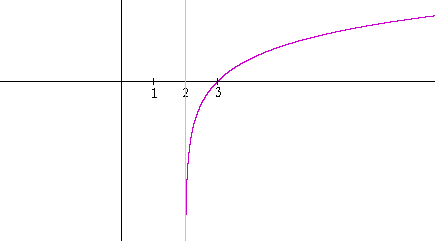Thank you for registering.

One of our academic counsellors will contact you within 1 working day.

Click to Chat

1800-5470-145

+91 7353221155

CART 0

• 0
MY CART (5)

Use Coupon: CART20 and get 20% off on all online Study Material

ITEM
DETAILS
MRP
DISCOUNT
FINAL PRICE
Total Price: Rs.

There are no items in this cart.
Continue Shopping

throw some light on logrithmic functionssuryakanth AskiitiansExpert-IITB
105 Points
11 years ago

Dear Yazdan,

THE LOGARITHMIC FUNCTION WITH BASE b  is the function

y  =  logb x.

b is normally a number greater than 1 (although it need only be greater than 0 and not equal to 1).  The function is defined for all x > 0.  Here is its graph for any base b.Note the following:

•  For any base, the x-intercept is 1.  Why?

The logarithm of 1 is 0.  y = logb1 = 0.

•  The graph passes through the point (b, 1).   Why?

The logarithm of the base is 1.  logbb = 1.

 • The graph is below the x-axis -- the logarithm is negative -- for 0 < x < 1. Which numbers are those that have negative logarithms?

Proper fractions.

 • The function is defined only for positive values of x. logb(−4), for example, makes no sense.  Since b is always positive, no power of b can produce a negative number.

•  The range of the function is all real numbers.

•  The negative y-axis is a vertical asymptote (Topic 18).

Example 1.   Translation of axes.   Here is the graph of the natural logarithm,  y = ln x  (Topic 20).And here is the graph of   y = ln (x − 2) -- which is its translation 2 units to the right.The x-intercept has moved from 1 to 3.  And the vertical asymptote has moved from 0 to 2.

Problem 1.   Sketch the graph of y = ln (x + 3).This is a translation 3 units to the left.   The x-intercept has moved from 1 to −2.  And the vertical asymptote has moved from 0 to −3.

All the best.

Win exciting gifts by answering the questions on Discussion Forum. So help discuss any query on askiitians forum and become an Elite Expert League askiitian.

Suryakanth –IITB